## Asymptotics of the maximal radius of an $L^r$-optimal sequence of quantizers

 Title Asymptotics of the maximal radius of an ${L}^r$-optimal sequence of quantizers Publication Type Miscellaneous Year of Publication 2008 Authors Gilles Pagès, and Abass Sagna Abstract Let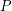be a probability distribution on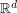(equipped with an Euclidean norm). Letand assume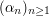is an (asymptotically)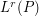-optimal sequence of-quantizers. In this paper we investigate the asymptotic behavior of the maximal radius sequence induced by the sequenceand defined to be for every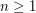,. We show that if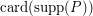is infinite, the maximal radius sequence goes to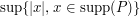asgoes to infinity. We then give the rate of convergence for two classes of distributions with unbounded support : distributions with exponential tails and distributions with polynomial tails.